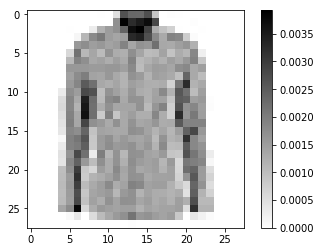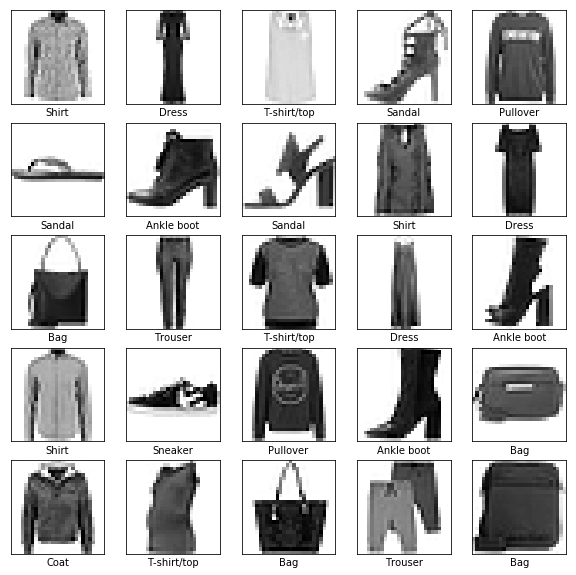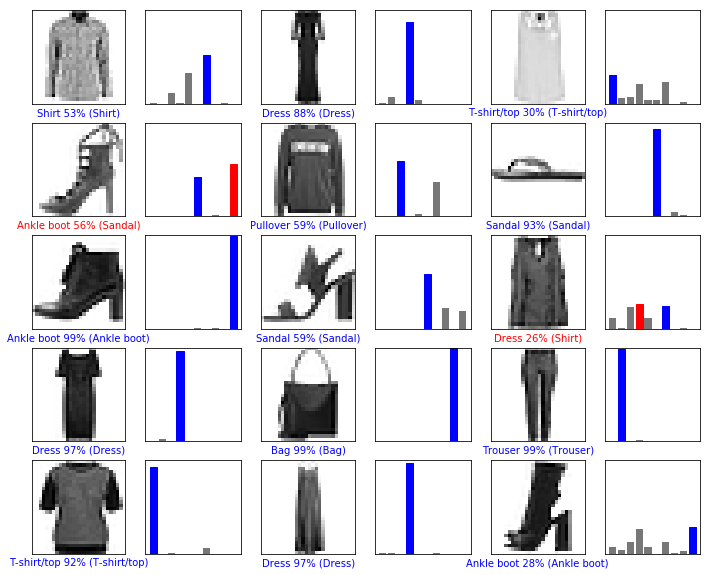!pip install -U tensorflow_datasets

Requirement already up-to-date: tensorflow_datasets in /srv/paws/lib/python3.6/site-packages
Requirement already up-to-date: termcolor in /srv/paws/lib/python3.6/site-packages (from tensorflow_datasets)
Requirement already up-to-date: wrapt in /srv/paws/lib/python3.6/site-packages (from tensorflow_datasets)
Requirement already up-to-date: future in /srv/paws/lib/python3.6/site-packages (from tensorflow_datasets)
Requirement already up-to-date: promise in /srv/paws/lib/python3.6/site-packages (from tensorflow_datasets)
Requirement already up-to-date: tqdm in /srv/paws/lib/python3.6/site-packages (from tensorflow_datasets)
Requirement already up-to-date: requests in /srv/paws/lib/python3.6/site-packages (from tensorflow_datasets)
Requirement already up-to-date: six in /srv/paws/lib/python3.6/site-packages (from tensorflow_datasets)
Requirement already up-to-date: protobuf>=3.6.1 in /srv/paws/lib/python3.6/site-packages (from tensorflow_datasets)
Requirement already up-to-date: absl-py in /srv/paws/lib/python3.6/site-packages (from tensorflow_datasets)
Requirement already up-to-date: typing>=3.6.4 in /srv/paws/lib/python3.6/site-packages (from promise->tensorflow_datasets)
Requirement already up-to-date: chardet<3.1.0,>=3.0.2 in /srv/paws/lib/python3.6/site-packages (from requests->tensorflow_datasets)
Requirement already up-to-date: idna<2.9,>=2.5 in /srv/paws/lib/python3.6/site-packages (from requests->tensorflow_datasets)
Requirement already up-to-date: urllib3<1.25,>=1.21.1 in /srv/paws/lib/python3.6/site-packages (from requests->tensorflow_datasets)
Requirement already up-to-date: certifi>=2017.4.17 in /srv/paws/lib/python3.6/site-packages (from requests->tensorflow_datasets)
Requirement already up-to-date: setuptools in /srv/paws/lib/python3.6/site-packages (from protobuf>=3.6.1->tensorflow_datasets)

"""
We'll build and train a neural network to classify images of clothing
Fashion MNIST dataset

and provides several sample datasets to work with. We're also using a few helper libraries.
!pip install -U tensorflow_datasets
"""

"\n We'll build and train a neural network to classify images of clothing \n Fashion MNIST dataset \n \n We'll need TensorFlow Datasets, an API that simplifies downloading and accessing datasets, \n and provides several sample datasets to work with. We're also using a few helper libraries.\n !pip install -U tensorflow_datasets\n"
!pip install tensorflow

Collecting tensorflow
Using cached https://files.pythonhosted.org/packages/77/63/a9fa76de8dffe7455304c4ed635be4aa9c0bacef6e0633d87d5f54530c5c/tensorflow-1.13.1-cp36-cp36m-manylinux1_x86_64.whl
Collecting tensorflow-estimator<1.14.0rc0,>=1.13.0 (from tensorflow)
Requirement already satisfied: termcolor>=1.1.0 in /srv/paws/lib/python3.6/site-packages (from tensorflow)
Requirement already satisfied: numpy>=1.13.3 in /srv/paws/lib/python3.6/site-packages (from tensorflow)
Collecting grpcio>=1.8.6 (from tensorflow)
Using cached https://files.pythonhosted.org/packages/f4/dc/5503d89e530988eb7a1aed337dcb456ef8150f7c06132233bd9e41ec0215/grpcio-1.19.0-cp36-cp36m-manylinux1_x86_64.whl
Collecting astor>=0.6.0 (from tensorflow)
Collecting tensorboard<1.14.0,>=1.13.0 (from tensorflow)
Using cached https://files.pythonhosted.org/packages/0f/39/bdd75b08a6fba41f098b6cb091b9e8c7a80e1b4d679a581a0ccd17b10373/tensorboard-1.13.1-py3-none-any.whl
Collecting gast>=0.2.0 (from tensorflow)
Using cached https://files.pythonhosted.org/packages/4e/35/11749bf99b2d4e3cceb4d55ca22590b0d7c2c62b9de38ac4a4a7f4687421/gast-0.2.2.tar.gz
Requirement already satisfied: absl-py>=0.1.6 in /srv/paws/lib/python3.6/site-packages (from tensorflow)
Requirement already satisfied: protobuf>=3.6.1 in /srv/paws/lib/python3.6/site-packages (from tensorflow)
Collecting wheel>=0.26 (from tensorflow)
Using cached https://files.pythonhosted.org/packages/96/ba/a4702cbb6a3a485239fbe9525443446203f00771af9ac000fa3ef2788201/wheel-0.33.1-py2.py3-none-any.whl
Collecting keras-applications>=1.0.6 (from tensorflow)
Using cached https://files.pythonhosted.org/packages/90/85/64c82949765cfb246bbdaf5aca2d55f400f792655927a017710a78445def/Keras_Applications-1.0.7-py2.py3-none-any.whl
Collecting keras-preprocessing>=1.0.5 (from tensorflow)
Using cached https://files.pythonhosted.org/packages/c0/bf/0315ef6a9fd3fc2346e85b0ff1f5f83ca17073f2c31ac719ab2e4da0d4a3/Keras_Preprocessing-1.0.9-py2.py3-none-any.whl
Requirement already satisfied: six>=1.10.0 in /srv/paws/lib/python3.6/site-packages (from tensorflow)
Collecting mock>=2.0.0 (from tensorflow-estimator<1.14.0rc0,>=1.13.0->tensorflow)
Using cached https://files.pythonhosted.org/packages/e6/35/f187bdf23be87092bd0f1200d43d23076cee4d0dec109f195173fd3ebc79/mock-2.0.0-py2.py3-none-any.whl
Collecting markdown>=2.6.8 (from tensorboard<1.14.0,>=1.13.0->tensorflow)
100% |████████████████████████████████| 92kB 2.9MB/s eta 0:00:01
Requirement already satisfied: werkzeug>=0.11.15 in /srv/paws/lib/python3.6/site-packages (from tensorboard<1.14.0,>=1.13.0->tensorflow)
Requirement already satisfied: setuptools in /srv/paws/lib/python3.6/site-packages (from protobuf>=3.6.1->tensorflow)
Collecting h5py (from keras-applications>=1.0.6->tensorflow)
Using cached https://files.pythonhosted.org/packages/30/99/d7d4fbf2d02bb30fb76179911a250074b55b852d34e98dd452a9f394ac06/h5py-2.9.0-cp36-cp36m-manylinux1_x86_64.whl
Collecting pbr>=0.11 (from mock>=2.0.0->tensorflow-estimator<1.14.0rc0,>=1.13.0->tensorflow)
Using cached https://files.pythonhosted.org/packages/14/09/12fe9a14237a6b7e0ba3a8d6fcf254bf4b10ec56a0185f73d651145e9222/pbr-5.1.3-py2.py3-none-any.whl
Building wheels for collected packages: gast
Running setup.py bdist_wheel for gast ... error
Complete output from command /srv/paws/bin/python3.6 -u -c "import setuptools, tokenize;__file__='/tmp/pip-build-6l6lcxdi/gast/setup.py';f=getattr(tokenize, 'open', open)(__file__);code=f.read().replace('\r\n', '\n');f.close();exec(compile(code, __file__, 'exec'))" bdist_wheel -d /tmp/tmpam862vd0pip-wheel- --python-tag cp36:
usage: -c [global_opts] cmd1 [cmd1_opts] [cmd2 [cmd2_opts] ...]
or: -c --help [cmd1 cmd2 ...]
or: -c --help-commands
or: -c cmd --help

error: invalid command 'bdist_wheel'

----------------------------------------
Failed building wheel for gast
Running setup.py clean for gast
Failed to build gast
Installing collected packages: pbr, mock, tensorflow-estimator, grpcio, astor, markdown, wheel, tensorboard, gast, h5py, keras-applications, keras-preprocessing, tensorflow
Running setup.py install for gast ... done
Successfully installed astor-0.7.1 gast-0.2.2 grpcio-1.19.0 h5py-2.9.0 keras-applications-1.0.7 keras-preprocessing-1.0.9 markdown-3.1 mock-2.0.0 pbr-5.1.3 tensorboard-1.13.1 tensorflow-1.13.1 tensorflow-estimator-1.13.0 wheel-0.33.1

from __future__ import absolute_import, division, print_function

# Import TensorFlow and TensorFlow Datasets
import tensorflow as tf
import tensorflow_datasets as tfds
tf.logging.set_verbosity(tf.logging.ERROR)

# Helper libraries
import math
import numpy as np
import matplotlib.pyplot as plt

# Improve progress bar display
import tqdm
import tqdm.auto
tqdm.tqdm = tqdm.auto.tqdm

WARNING: The TensorFlow contrib module will not be included in TensorFlow 2.0.
* https://github.com/tensorflow/community/blob/master/rfcs/20180907-contrib-sunset.md
If you depend on functionality not listed there, please file an issue.


print(tf.__version__)

# This will go away in the future.
# If this gives an error, you might be running TensorFlow 2 or above
# If so, the just comment out this line and run this cell again
tf.enable_eager_execution()

1.13.1

dataset, metadata = tfds.load('fashion_mnist', as_supervised=True, with_info=True)
train_dataset, test_dataset = dataset['train'], dataset['test']

"""
The images are 28  ×  28 arrays, with pixel values in the range [0, 255]. The labels are an array of integers,
in the range [0, 9]. These correspond to the class of clothing the image represents:

0 - > t-shirt, 1-> trouser and so son
"""

'\nThe images are 28  ×  28 arrays, with pixel values in the range [0, 255]. The labels are an array of integers, \nin the range [0, 9]. These correspond to the class of clothing the image represents:\n\n0 - > t-shirt, 1-> trouser and so son\n'
class_names = ['T-shirt/top', 'Trouser', 'Pullover', 'Dress', 'Coat',
'Sandal',      'Shirt',   'Sneaker',  'Bag',   'Ankle boot']

num_train_examples = metadata.splits['train'].num_examples
print("Number of training examples: {}".format(num_train_examples))
print("Number of test examples:     {}".format(num_test_examples))

Number of training examples: 60000
Number of test examples:     10000

"""
We need to have every pixel value to be a number between 0 and 1
"""
def normalize(images, labels):
images = tf.cast(images, tf.float32)
images /= 255
return images, labels

# The map function applies the normalize function to each element in the train
# and test datasets
train_dataset =  train_dataset.map(normalize)
test_dataset  =  test_dataset.map(normalize)

print(test_dataset.take(1))

<DatasetV1Adapter shapes: ((28, 28, 1), ()), types: (tf.float32, tf.int64)>

# Take a single image, and remove the color dimension by reshaping
for image, label in test_dataset.take(1):
break
image = image.numpy().reshape((28,28))

# Plot the image - voila a piece of fashion clothing
plt.figure()
plt.imshow(image, cmap=plt.cm.binary)
plt.colorbar()
plt.grid(False)
plt.show()plt.figure(figsize=(10,10))
i = 0
for (image, label) in test_dataset.take(25):
image = image.numpy().reshape((28,28))
plt.subplot(5,5,i+1)
plt.xticks([])
plt.yticks([])
plt.grid(False)
plt.imshow(image, cmap=plt.cm.binary)
plt.xlabel(class_names[label])
i += 1
plt.show()"""
The basic building block of a neural network is the layer. A layer extracts a representation
from the data fed into it.
Hopefully, a series of connected layers results in a representation that
is meaningful for the problem at hand.

Much of deep learning consists of chaining together simple layers.
Most layers, like tf.keras.layers.Dense, have internal parameters which
"""

'\nThe basic building block of a neural network is the layer. A layer extracts a representation \nfrom the data fed into it. \nHopefully, a series of connected layers results in a representation that \nis meaningful for the problem at hand.\n\nMuch of deep learning consists of chaining together simple layers. \nMost layers, like tf.keras.layers.Dense, have internal parameters which \nare adjusted ("learned") during training.\n'
model = tf.keras.Sequential([
tf.keras.layers.Flatten(input_shape=(28, 28, 1)),
tf.keras.layers.Dense(128, activation=tf.nn.relu),
# these are the 10 probabilities for the different clothing items, they sum up to 1
tf.keras.layers.Dense(10,  activation=tf.nn.softmax)
])

"""
Before the model is ready for training, it needs a few more settings. These are added during the model's compile step:

Loss function — An algorithm for measuring how far the model's outputs are from the desired output.
The goal of training is this measures loss.
Optimizer —An algorithm for adjusting the inner parameters
of the model in order to minimize loss.
Metrics —Used to monitor the training and testing steps.
The following example uses accuracy, the fraction of the images that are correctly classified.
"""

"\nBefore the model is ready for training, it needs a few more settings. These are added during the model's compile step:\n\nLoss function — An algorithm for measuring how far the model's outputs are from the desired output. \nThe goal of training is this measures loss.\nOptimizer —An algorithm for adjusting the inner parameters \nof the model in order to minimize loss.\nMetrics —Used to monitor the training and testing steps. \nThe following example uses accuracy, the fraction of the images that are correctly classified.\n"
model.compile(optimizer='adam',
loss='sparse_categorical_crossentropy',
metrics=['accuracy'])

"""
First, we define the iteration behavior for the train dataset:

Repeat forever by specifying dataset.repeat() (the epochs parameter described below limits how
long we perform training).
The dataset.shuffle(60000) randomizes the order so our model cannot learn anything from the order of the examples.
And dataset.batch(32) tells model.fit to use batches of 32 images and labels when updating the model variables.
Training is performed by calling the model.fit method:

Feed the training data to the model using train_dataset.
The model learns to associate images and labels.
The epochs=5 parameter limits training to 5 full iterations of the training dataset,
so a total of 5 * 60000 = 300000 examples.
"""

BATCH_SIZE = 32
train_dataset = train_dataset.repeat().shuffle(num_train_examples).batch(BATCH_SIZE)
test_dataset = test_dataset.batch(BATCH_SIZE)
model.fit(train_dataset, epochs=5, steps_per_epoch=math.ceil(num_train_examples/BATCH_SIZE))

Epoch 1/5
1875/1875 [==============================] - 54s 29ms/step - loss: 1.0707 - acc: 0.6592
Epoch 2/5
1875/1875 [==============================] - 13s 7ms/step - loss: 0.6315 - acc: 0.7739
Epoch 3/5
1875/1875 [==============================] - 12s 6ms/step - loss: 0.5513 - acc: 0.8044
Epoch 4/5
1875/1875 [==============================] - 13s 7ms/step - loss: 0.5086 - acc: 0.8216
Epoch 5/5
1875/1875 [==============================] - 13s 7ms/step - loss: 0.4867 - acc: 0.8290

<tensorflow.python.keras.callbacks.History at 0x7f419466d7b8>
"""
Next, compare how the model performs on the test dataset.
Use all examples we have in the test dataset to assess accuracy.
"""

test_loss, test_accuracy = model.evaluate(test_dataset, steps=math.ceil(num_test_examples/32))
print('Accuracy on test dataset:', test_accuracy)

313/313 [==============================] - 1s 4ms/step - loss: 0.4990 - acc: 0.8222
Accuracy on test dataset: 0.8222

"""
Now lest's test predictions
"""
for test_images, test_labels in test_dataset.take(1):
test_images = test_images.numpy()
test_labels = test_labels.numpy()
predictions = model.predict(test_images)

predictions.shape

# let's see one of the predictions, A prediction is an array of 10 numbers.
print("array of predictions for item 0",predictions)

# let's test that teh label with highest probability is the one we expect
print("true label for item 0",test_labels)

array of predictions for item 0 [6.6813696e-03 1.2119212e-03 1.1651280e-01 9.0496698e-03 3.3437991e-01
1.1685527e-05 5.2636534e-01 5.2139143e-08 5.7870187e-03 1.6664472e-07]
true label for item 0 6

# let's graph the predictions for test set
#  Correct prediction labels are blue and incorrect prediction labels are red.
def plot_image(i, predictions_array, true_labels, images):
predictions_array, true_label, img = predictions_array[i], true_labels[i], images[i]
plt.grid(False)
plt.xticks([])
plt.yticks([])

plt.imshow(img[...,0], cmap=plt.cm.binary)

predicted_label = np.argmax(predictions_array)
if predicted_label == true_label:
color = 'blue'
else:
color = 'red'

plt.xlabel("{} {:2.0f}% ({})".format(class_names[predicted_label],
100*np.max(predictions_array),
class_names[true_label]),
color=color)

def plot_value_array(i, predictions_array, true_label):
predictions_array, true_label = predictions_array[i], true_label[i]
plt.grid(False)
plt.xticks([])
plt.yticks([])
thisplot = plt.bar(range(10), predictions_array, color="#777777")
plt.ylim([0, 1])
predicted_label = np.argmax(predictions_array)

thisplot[predicted_label].set_color('red')
thisplot[true_label].set_color('blue')

# Plot the first X test images, their predicted label, and the true label
# Color correct predictions in blue, incorrect predictions in red
num_rows = 5
num_cols = 3
num_images = num_rows*num_cols
plt.figure(figsize=(2*2*num_cols, 2*num_rows))
for i in range(num_images):
plt.subplot(num_rows, 2*num_cols, 2*i+1)
plot_image(i, predictions, test_labels, test_images)
plt.subplot(num_rows, 2*num_cols, 2*i+2)
plot_value_array(i, predictions, test_labels)